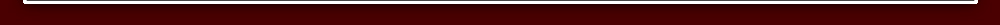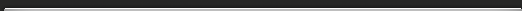ALT Codes
 §To obtain an ALT Character: 1.Make certain that the Num Lock key has been pressed to activate the numeric key section of the keyboard. 2. Depress the Alt key. 3.While the Alt key is depressed, type the proper sequence of numbers (on the numeric keypad) of the ALT code from the table above. 4.Release the Alt key, and the character will appear. 5.On a laptop computer, there usually is no numeric keypad. So, instead, depress the Fn (function) key, then the Alt key, then the numerical code sequence. The numbers are on the fronts of certain keyboard keys: M=0, J=1, K=2, L=3, U=4, I=5, O=6, 7=7, 8=8, and 9=9.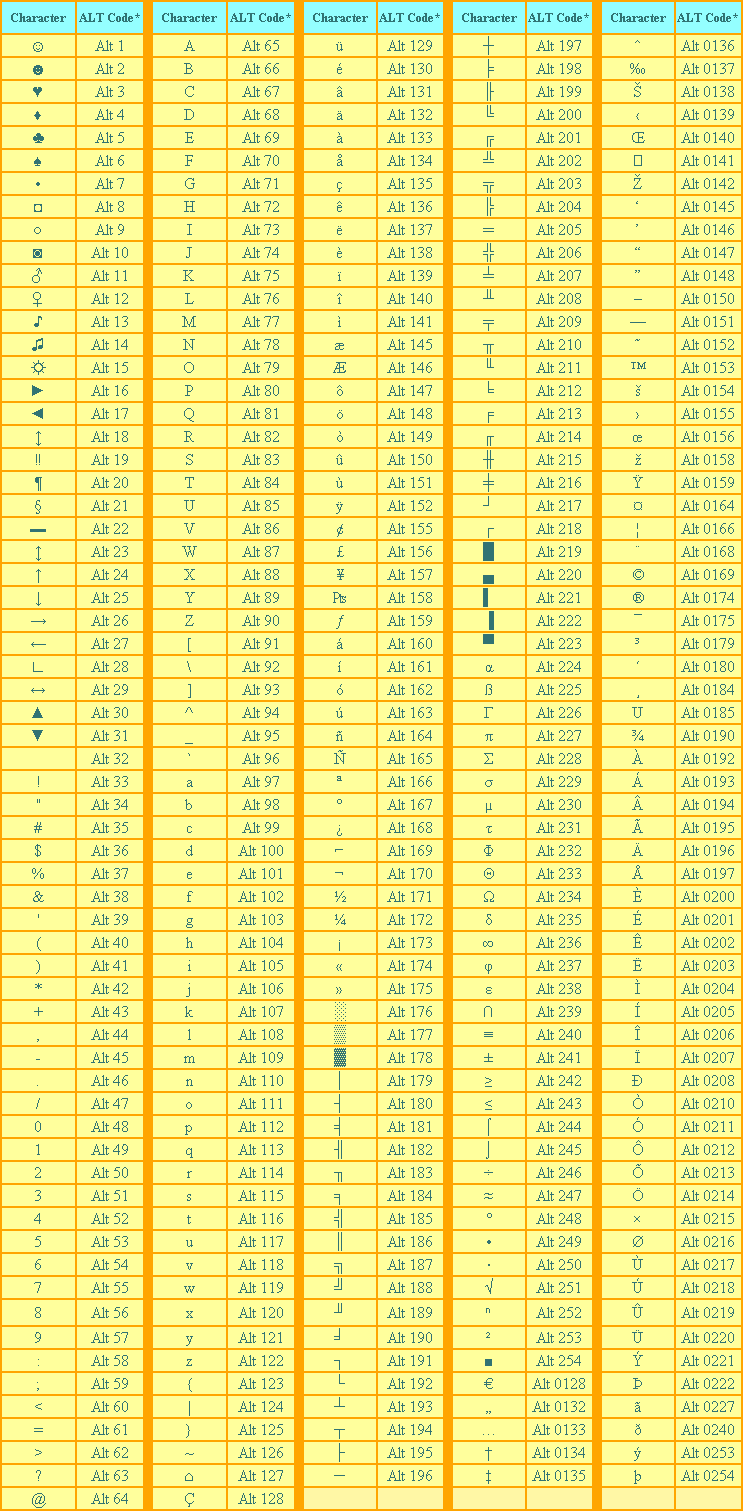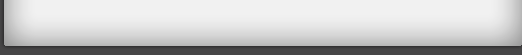JavedzzPromote Your Page Too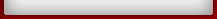Your Ad Here